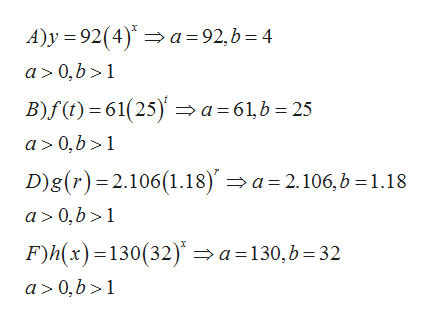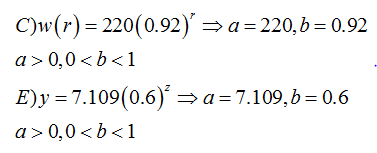# Which of the following exponential functions represent growth and which represent decay?a.   y=92⋅4x represents exponential  b. f(t)=61⋅(25)t represents exponential  c. w(r)=220⋅0.92r represents exponential  d.   g⁢(r)=2⋅106⋅1.18r represents exponential  e.   y=7⋅109⋅0.6z represents exponential  f. h(x)=130⋅(32)x represents exponential

Question
31 views

Which of the following exponential functions represent growth and which represent decay?

a.   y=92⋅4x represents exponential

b. f(t)=61⋅(25)t represents exponential

c. w(r)=220⋅0.92r represents exponential

d.   g⁢(r)=2⋅106⋅1.18r represents exponential

e.   y=7⋅109⋅0.6z represents exponential

f. h(x)=130⋅(32)x represents exponential

check_circle

Step 1

The exponential function f(x)=a(b)^x represents

a) growth if a>0 and b>1

b) decay if a>0 and 1<b<1

Step 2

We compare the given function by f(x)=a(b)^x and find a and b

In case A, B,D and F , a>0 and b>1, so the functions represent growthhelp_outlineImage TranscriptioncloseA)y 92(4)a=92,b 4 a 0, b >1 B)f() 61(25)a 61,b 25 a 0,b>1 D)g(r) 2.106(1.18)' a 2.106, b 1.18 a 0,b 1 F)h(x) 130(32)a130,b 32 a> 0,b>1 fullscreen
Step 3

In case C and E , a>0 and 0<b<1, so the functions represent decay...

### Want to see the full answer?

See Solution

#### Want to see this answer and more?

Solutions are written by subject experts who are available 24/7. Questions are typically answered within 1 hour.*

See Solution
*Response times may vary by subject and question.
Tagged in

### Exponents